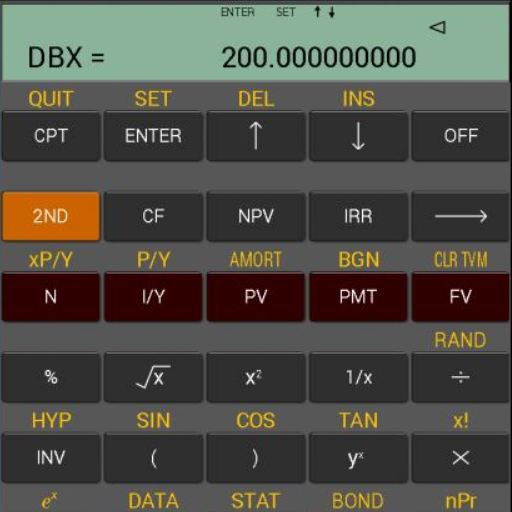Ba Financial Calculator plus

基本信息

• ASIN: B07XCCC752
• 最初的发行日期（第一版）: 2019年9月6日
• 开发者最新更新: 2019年9月6日
• 分级:
• 用户评分:

商品特性

• Scientific calculation
• Time Value of Money calculations(TVM)
• Amortization Schedule
• Cash Flow analysis,
• Bond calculations
• Depreciation calculations
• Statistics Analysis
• Percent Change/Compound Interest
• Interest Conversion .
• Date calculations.

商品描述

This finance calculator app for Android devices is very similar as BA II Plus Professional Financial Calculator.

This finance calculator app has the same usage and operation sequences with BA II Plus Professional Financial

Calculator, and it provide scientific calculation function and financial calculation such as time-value-of-money,

amortization schedule, cash flow, and so on. This app uses easier natural input sequences than RPN used by HP 12C Financial

Calculator.

******Key Features ******
• Vibrate and change color when pressing button,and turn on or turn off vibration in format worksheet by set VIB-ON or

VIB-OFF.
• Support dates format and number separators format of USA and European, Support degrees and radians of the Angle Units.
• Scientific calculation(algebraic operating system(AOS), chain calculation method(Chn)): universal power, combinations,

permutations, square, square root, reciprocal, sine, arcsine, hyperbolic sine, hyperbolic arcsine.
• Time Value of Money calculations(TVM), Number of periods(N), Interest rate per year(I/Y), Present value(PV), Payment

(PMT), Future value(FV),and so on.
• Amortization Schedule, Starting payment(P1), Ending payment(P2), Balance(BAL), Principal paid(PRN), Interest paid(INT).
• Cash Flow analysis, support up to 99 data pairs input, Net Present Value (NPV), and Internal Rate of Return (IRR), Net

Future Value (NFV), Payback (PB), Discounted Payback (DPB), Reinvestment Rate (RI), and Modified Internal Rate of Return

(MOD).
• Bond calculations, bond price(PRI), yield to maturity or call(YLD), accrued interest(AI), modified duration(DUR).
• Depreciation calculations, support 6 different methodologies, Depreciation for the year(DEP), Remaining book value at

the end of the year(RBV), Remaining depreciable value(RDV)
• Statistics Analysis, support up to 99 data pairs input, Support 5 analysis models(Standard linear regression,

Logarithmic regression, Exponential regression, Power regression, One-variable statistics).
• Percent Change/Compound Interest
• Interest Conversion
• Date calculations,
• Profit Margin,
• Breakeven
• 10 Memories for storage
• Automatically save/restore all format settings during app close/start.
• More……

******Special explanation ******
This finance calculator support android 2.1 or above, and the screen size of android device above 3.5’ have better effect

.

******Support ******
If you do not know how to use it, you can refer to the user manual of BA II Plus Professional Financial Calculator, or

email to liningbo_lnb@aliyun.com to ask for user manual.
If you have suggestions or find bug ,please send you advice or test case to liningbo_lnb@aliyun.com, Thank you.

无顾客评论

 5 星 (0%) 0% 4 星 (0%) 0% 3 星 (0%) 0% 2 星 (0%) 0% 1 星 (0%) 0%